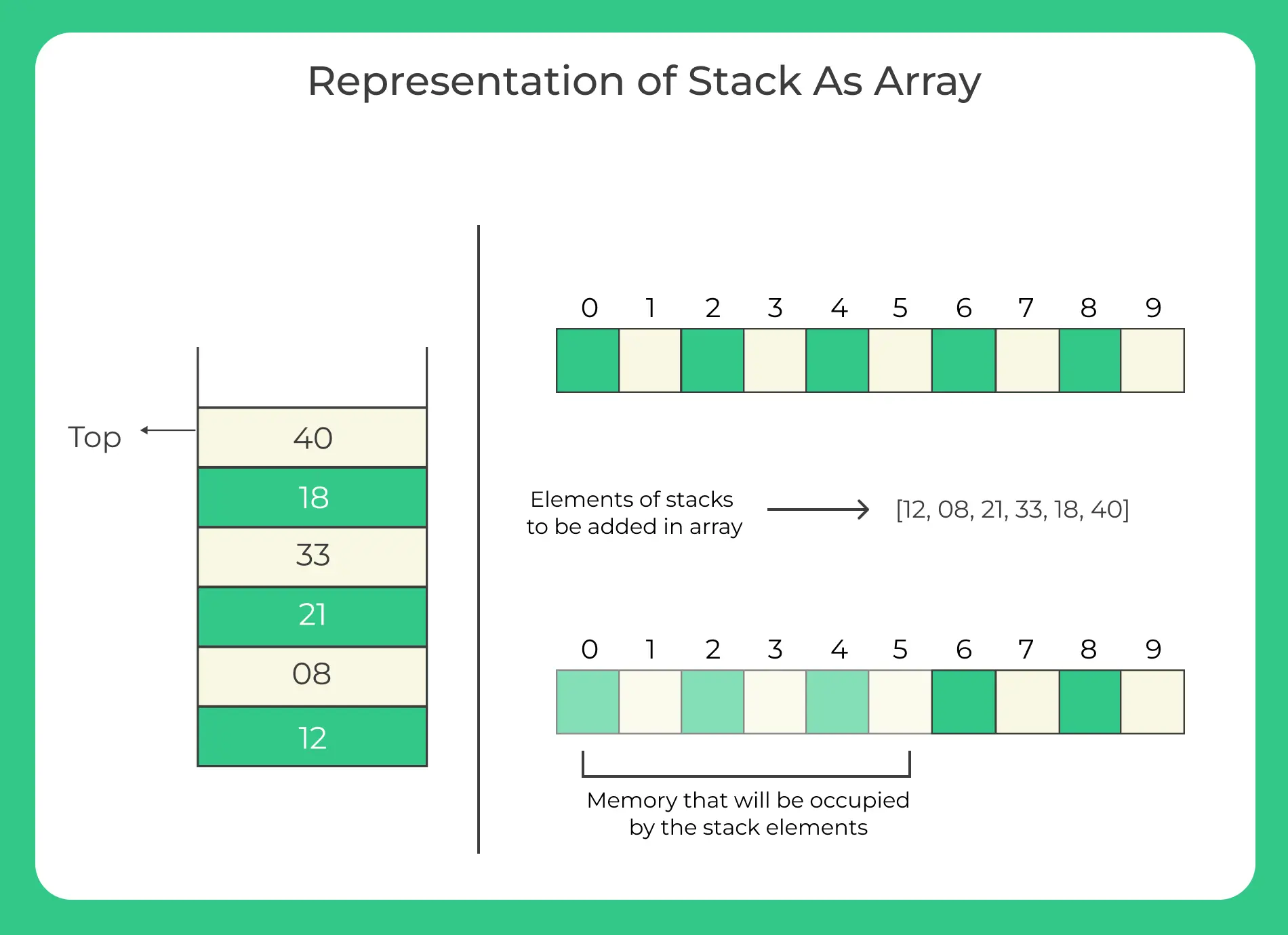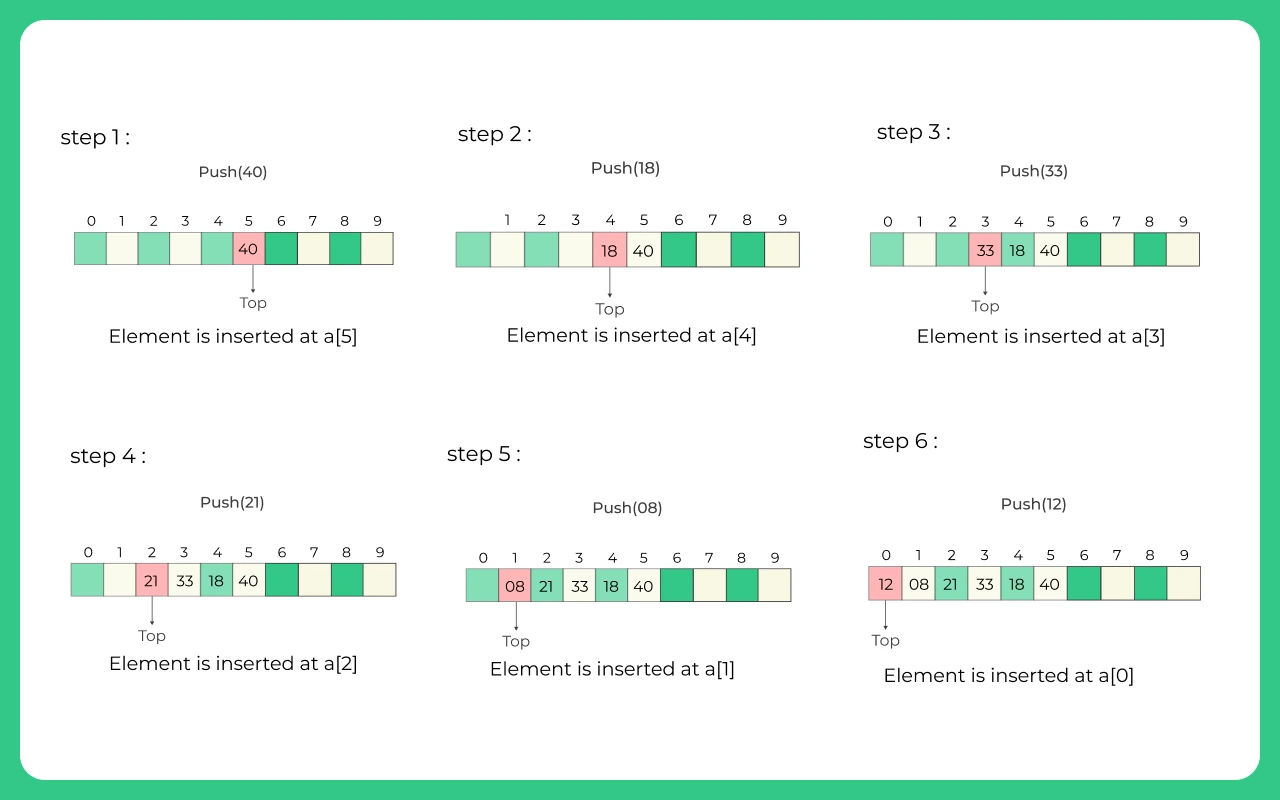# Stack using an array in C++

Stack is one of the most important linear data structures, that we can use in different algorithms or implement the application in various problem solving. A stack is a linear data structure, that means it can be easily implememented using an array. You can use array to store some elements in the specific order you recieve them. Then you can use simple easy techniques to manage the data so that it can work like an Stack. Here we will go through the Representation of a Stack using Array.### What is Stack?

Stack is a data structure where you can add any kind of and store then in a LIFO (last in first out) manner. To kno more about Stack in C++ STL :

Stack in C++ STL

suppose you push 2,3,4 in to the stack respectively. When you pop out a value, the last pushed value (4) will be deleted from it. Then if you try to see what is in the top of the stack, you will find its the second last value entered (2)

### Representation using an Array

There are some major differences between a stack and an array. like,

• Array size is fixed, but the same isn’t applied for stack.
• Also, in a stack you can only pop or push values only from or in the last element

Now let us focus on how we can implement a stack using an array.

#### For Example:

We are given a stack of elements:  12 , 08 , 21 , 33 , 18 , 40.## Code for Stack in C++ (using Class)

Run
```// Cpp Program for Implmentation of stack (array) using structure
#include<bits/stdc++.h>
using namespace std;

class Stack
{
public:
int top;
int maxSize;
int* array;
Stack(int max)
{
top=-1;
maxSize=max;
array=new int[max];
}
int isFull()
{
if(top==maxSize-1)
cout<<"Will not be able to push maxSize reached"<<endl;
}

int isEmpty()
{
if(top==-1)
cout<<"Will not be able to pop minSize reached"<<endl;
}
void push(int item)
{
if(isFull()) return;
array[++top]=item;
cout<<"We have pushed "<<item<<" to stack"<<endl;
}
int pop()
{
if(isEmpty()) return INT_MIN;
return array[top--];
}
int peek()
{
if(isEmpty()) return INT_MIN;
return array[top];
}
};

int main()
{
Stack stack(10);
stack.push(5);
stack.push(10);
stack.push(15);
int flag=1;
while(flag)
{
if(!stack.isEmpty())
cout<<"We have popped "<< stack.pop()<<" from stack"<<endl;
else
cout<<"Can't Pop stack must be empty\n";

flag=0;
}

}```

### O/P

```We have pushed 5 to stack
We have pushed 10 to stack
We have pushed 15 to stack
We have popped 15 from the stack
```

### Related Banners

Get PrepInsta Prime & get Access to all 200+ courses offered by PrepInsta in One Subscription

### Stacks

• Introduction to Stack in Data Structure
• Operations on a Stack
• Stack: Infix, Prefix and Postfix conversions
• Stack Representation in –
C | C++ | Java
• Representation of a Stack as an Array. –
C | C++ | Java
• Representation of a Stack as a Linked List. –
C | C++ | Java
• Infix to Postfix Conversion –
C | C++ | Java
• Infix to prefix conversion in –
C | C++ | Java
• Postfix to Prefix Conversion in –
C | C++ | Java

### Queues

• Queues in Data Structures (Introduction)
• Queues Program in C and implementation
• Implementation of Queues using Arrays | C Program
• Types of Queues in Data Structure
• Application of Queue Data Structure
• Insertion in Queues Program (Enqueuing) –
C | C++ | Java
• Deletion (Removal) in Queues Program(Dequeuing) –
C | C++ | Java
• Reverse a Queue –
C | C++ | Java
• Queues using Linked Lists –
C | C++ | Java
• Implement Queue using Stack –
C | C++ | Java
• Implement Queue using two Stacks –
C | C++ | Java

### Priority Queue

• Application of Priority Queue
• Priority Queue Example
• Priority Queue Introduction –
C | C++ | Java
• Priority Queue Implementation using Array –
C | C++ | Java
• Priority Queue using Linked List –
C | C++ | Java
• Priority Queue Insertion and Deletion-
C | C++ | Java

### Priority Queue

• Application of Priority Queue
• Priority Queue Example
• Priority Queue Introduction – C | C++ | Java
• Priority Queue Implementation using Array – C | C++ | Java
• Priority Queue using Linked List – C | C++ | Java
• Priority Queue Insertion and Deletion- C | C++ | Java